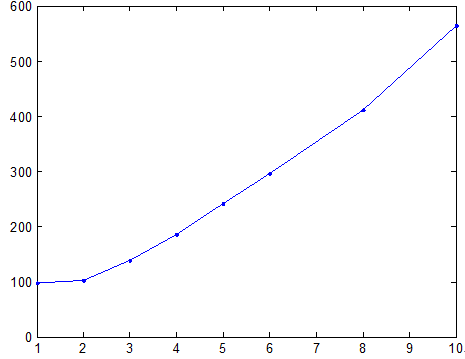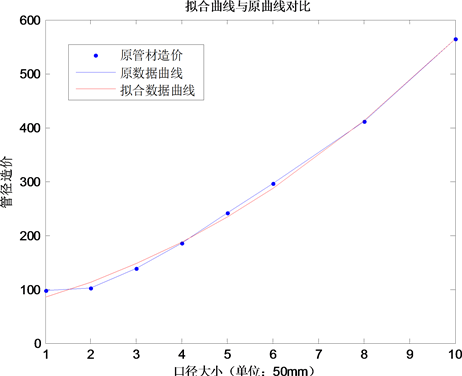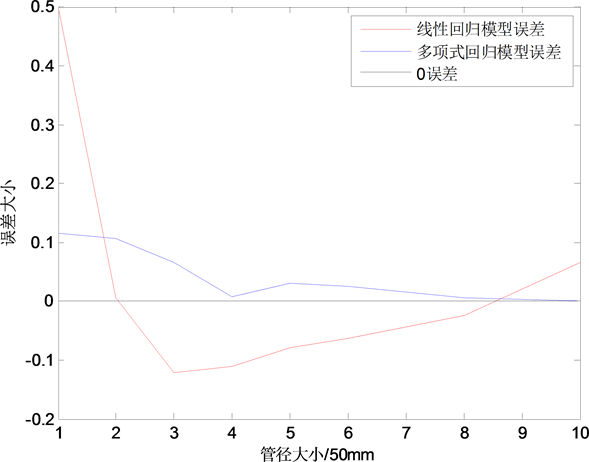# 给排水管道工程中管材造价的确定Determination of Cost of Pipe Material in Water Supply and Drainage Pipework

DOI: 10.12677/SA.2018.73035, PDF, HTML, XML, 下载: 623  浏览: 990  科研立项经费支持

Abstract: The relationship between cost of pipe material and pipe size in water supply and drainage pipework is studied in this paper. In order to determine the cost formula of pipe material, we established a liner regression and one-dimensional polynomial regression model and fitted the coefficient of model function by least squares. The effectiveness of the algorithm is verified by numerical experiments.

1. 引言

2. 求解模型建立

$M=\underset{i=1}{\overset{t}{\sum }}{q}_{i}=\underset{i=1}{\overset{t}{\sum }}{p}_{i}{y}_{i}$Table 1. Price list of unit cost and diameter of pipe material

2.1. 一元线性回归模型

$\left\{\begin{array}{l}y=a+bx+\epsilon \\ E\left(\epsilon \right)=0,D\left(\epsilon \right)={\sigma }^{2}\end{array}$

$\left\{\begin{array}{l}{y}_{i}=a+b{x}_{i}+{\epsilon }_{i},i=1,2,\cdots ,n\\ E\left({\epsilon }_{i}\right)=0,D\left({\epsilon }_{i}\right)={\sigma }^{2},{\epsilon }_{i}>0\end{array}$

$Q=Q\left(a,b\right)=\underset{i=1}{\overset{n}{\sum }}{\epsilon }^{2}=\underset{i=1}{\overset{n}{\sum }}{\left({y}_{i}-a-b{x}_{i}\right)}^{2}$ (1)

$a,b$ 的估计值 $\stackrel{^}{a},\stackrel{^}{b}$ ，使得

$Q\left(\stackrel{^}{a},\stackrel{^}{b}\right)={Q}_{\mathrm{min}}\left(a,b\right)$ (2)

$\left\{\begin{array}{l}\underset{i=1}{\overset{n}{\sum }}\left({y}_{i}-\stackrel{^}{a}-\stackrel{^}{b}{x}_{i}\right)=0\\ \underset{i=1}{\overset{n}{\sum }}{x}_{i}\left({y}_{i}-\stackrel{^}{a}-\stackrel{^}{b}{x}_{i}\right)=0\end{array}$ (3)

$\left\{\begin{array}{l}\stackrel{^}{a}=\stackrel{¯}{y}-\stackrel{^}{b}\stackrel{¯}{x}\\ \stackrel{^}{b}=\frac{\stackrel{¯}{xy}-\stackrel{¯}{x}\text{ }\stackrel{¯}{y}}{\stackrel{¯}{{x}^{2}}-{\stackrel{¯}{x}}^{2}}\end{array}$ (4)

$y=\stackrel{^}{a}+\stackrel{^}{b}x,\stackrel{¯}{y}=\stackrel{^}{b}\left(x-\stackrel{¯}{x}\right)$

2.2. 一元多项式回归

$f\left(x\right)=\underset{j=0}{\overset{m}{\sum }}{a}_{j}{x}^{j}$ (5)

${P}_{\mathrm{min}}=\underset{i=1}{\overset{n}{\sum }}{\left[f\left({x}_{i}\right)-{y}_{i}\right]}^{2}=\underset{i=1}{\overset{n}{\sum }}{\left(\underset{j=0}{\overset{m}{\sum }}{a}_{j}{x}^{j}-{y}_{i}\right)}^{2}$ (6)

$\frac{\partial P}{\partial {a}_{j}}=2\underset{i=1}{\overset{n}{\sum }}\left(\underset{k=0}{\overset{m}{\sum }}{a}_{k}{x}_{i}^{k}-{y}_{i}\right){x}_{i}^{j}\text{\hspace{0.17em}}\text{\hspace{0.17em}}\text{\hspace{0.17em}}\left(j=0,1,2,\cdots ,m\right)$ (7)

$\frac{\partial P}{\partial {a}_{j}}=2\underset{i=1}{\overset{n}{\sum }}\left(\underset{k=0}{\overset{m}{\sum }}{a}_{k}{x}_{i}^{k}-{y}_{i}\right){x}_{i}^{j}=0$ (8)

$\underset{k=0}{\overset{m}{\sum }}\left(\underset{i=i}{\overset{n}{\sum }}{x}_{i}^{j+k}\right){a}_{k}=\underset{i=i}{\overset{n}{\sum }}{x}_{i}^{j}{y}_{i}\text{}\left(j=0,1,2,\cdots ,m\right)$ (9)

$=\left[\begin{array}{cccc}{c}_{0}& {c}_{1}& \cdots & {c}_{m}\\ {c}_{1}& {c}_{2}& \cdots & {c}_{m+1}\\ ⋮& ⋮& \ddots & ⋮\\ {c}_{m}& {c}_{m+1}& \cdots & {c}_{2m}\end{array}\right]×\left[\begin{array}{c}{a}_{0}\\ {a}_{1}\\ ⋮\\ {a}_{m}\end{array}\right]=\left[\begin{array}{c}{b}_{0}\\ {b}_{1}\\ ⋮\\ {b}_{m}\end{array}\right]$ (10)

$\left\{\begin{array}{l}{c}_{k}=\underset{i=i}{\overset{n}{\sum }}{x}_{i}^{k},\left(k=0,1,2,\cdots ,m\right)\\ {b}_{k}=\underset{i=i}{\overset{n}{\sum }}{x}_{i}^{k}{y}_{i},\left(k=0,1,2,\cdots ,m\right)\end{array}$ (11)

$C=\left[\begin{array}{cccc}{c}_{0}& {c}_{1}& \cdots & {c}_{m}\\ {c}_{1}& {c}_{2}& \cdots & {c}_{m+1}\\ ⋮& ⋮& \ddots & ⋮\\ {c}_{m}& {c}_{m+1}& \cdots & {c}_{2m}\end{array}\right]$$A=\left[\begin{array}{c}{a}_{0}\\ {a}_{1}\\ ⋮\\ {a}_{m}\end{array}\right]$$B=\left[\begin{array}{c}{b}_{0}\\ {b}_{1}\\ ⋮\\ {b}_{m}\end{array}\right]$ ，(10)式可以表示为： $CA=B$ 。解出多项式回归的系数 ${a}_{j}\left(j=0,1,2,\cdots ,m\right)$ 就能够得到拟合函数关系：

$f\left(x\right)={a}_{0}+{a}_{1}x+\cdots +{a}_{m}{x}^{m}=\underset{j=0}{\overset{m}{\sum }}{a}_{j}{x}^{j}$

2.3. 模型比较

$f\left(x\right)={a}_{0}+{a}_{1}x+\cdots +{a}_{m}{x}^{m}$

3. 数值算例

3.1. 具体求解过程

1) 做出散点图

2) 确定数学模型

① 一元线性回归模型。首先设 ${y}_{i}=a+b{x}_{i}+{\epsilon }_{i},i=1,2,\cdots ,n$ 再设

${Q}_{\mathrm{min}}=Q\left(a,b\right)=\underset{i=1}{\overset{n}{\sum }}{\epsilon }_{i}^{2}=\underset{i=1}{\overset{n}{\sum }}\left({y}_{i}-a-b{x}_{i}^{2}\right)$Table 2. Price list of unit cost and diameter of pipe for graphite cast iron pipesFigure 1. The scatter point diagram of pipe cost and pipe diameter

$\left\{\begin{array}{l}\stackrel{^}{a}=\stackrel{¯}{y}-\stackrel{^}{b}\stackrel{¯}{x}\\ \stackrel{^}{b}=\frac{\stackrel{¯}{xy}-\stackrel{¯}{x}\text{ }\stackrel{¯}{y}}{\stackrel{¯}{{x}^{2}}-{\stackrel{¯}{x}}^{2}}\end{array}$

$\left\{\begin{array}{l}\stackrel{^}{a}=-4.1816\\ \stackrel{^}{b}=53.0580\end{array}$

② 多项式回归模型

$C=\left[\begin{array}{ccc}8& 39& 255\\ 39& 255& 1953\\ 255& 1953& 16371\end{array}\right]$$B=\left[\begin{array}{c}2035.8\\ 13367\\ 104080\end{array}\right]$

${C}^{-1}=\left[\begin{array}{ccc}1.4610& -0.5694& 0.0452\\ -0.5694& 0.2673& -0.0230\\ 0.0452& -0.0230& 0.0021\end{array}\right]$

$A={C}^{-1}B=\left[\begin{array}{c}64.4780\\ 18.0544\\ 3.1994\end{array}\right]$

${a}_{0}=64.4780,{a}_{1}=18.0544,{a}_{2}=3.1994$ 拟合的函数为

$f\left(x\right)=64.4780+18.0544x+3.1994{x}^{2}$

3.2. 误差对比分析Figure 2. Comparison of two kinds of fitting function values with original dataFigure 3. Numerical error contrast diagram of two models

3.3. 模型代入求解

$M=\underset{i=1}{\overset{t}{\sum }}{p}_{i}\left(64.4780+18.0544x+3.1994{x}^{2}\right)$

  陈健. 新型塑料给排水管材的特点及应用前景[J]. 山西建筑, 2007, 33(32): 168-170.  吴亚群. 树状给水管网的优化设计研究[D]: [硕士学位论文]. 西安: 长安大学, 2014.  刘瑞, 姚青云, 李铁军. U-PVC管材造价数值预测模型的研究[J]. 宁夏工程技术, 2011, 10(2): 164-166.  赵静, 但琦. 数学建模与数学实验[M]. 北京: 高等教育出版社, 2003: 260-285.  余亚冰, 罗磊, 于波. 大型净水厂自动投药系统的建模与研究[J]. 甘肃科学学报, 2012, 24(2): 120-123.  白丹. 给水输配水管网系统优化设计研究[D]: [博士学位论文]. 西安: 西安理工大学, 2003.